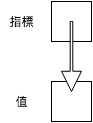#[Rust] 程式設計教學：所有權 (Ownership)

## 指標與參考``````#include <stdio.h>

int main(void) {
int n = 5;

// Get the address of n
int* n_ptr = &n;

// Dereference n_ptr to get n
printf("%d\n", *n_ptr);

return 0;
}``````

``````fn main() {
let n = 5;

// Get the reference of n
let n_ref = &n;  // & i32

// Dereference n_ref to get n
println!("{}", *n_ref);
}``````

``````#include <vector>
#include <iostream>

using std::vector;
using std::cout;
using std::endl;

int main() {
vector<int> vec {1, 2, 3};

// Modify vec in-place!
for (auto ptr = vec.begin(); ptr != vec.end(); ptr++) {
*ptr = (*ptr) * (*ptr);
}

// Print out the data of vec in console
for (auto ptr = vec.begin(); ptr != vec.end(); ptr++) {
cout << *ptr << " ";
}
cout << endl;

return 0;
}``````

``````fn main() {
let mut vec = vec![1, 2, 3];

// Modify vec in-place!
for item in vec.iter_mut() {
*item = (*item) * (*item);
}

// Print out the data of vec in console
println!("{:?}", vec);
}``````

## 所有權 (Ownership)

C 或 C++ 給使用者較大的自由，但對某些指標使用造成的問題，沒有特定的規範。我們來看一個 C 語言的迷途指標 (dangling pointer) 的例子：

``````#include <stdio.h>
#include <stdlib.h>

int main() {
const int SIZE = 10;

// Allocate memory for the array a.
int* a = (int*) malloc(SIZE * sizeof(int));

// Set the value in a
for (int i = 0; i < SIZE; i++) {
int j = i + 1;
a[i] = j * j;
}

// Share the address of a to a1
int* a1 = a;

// Oh, the memory of a is freed!
free(a);
a = NULL;

/* Now a1 becomes dangling pointer.
Accessing data via a dangling pointer is
an undefined behavior. */
printf("%d\n", a1);

return 0;
}``````

``````fn main() {
let v = vec![1, 2, 3];

// Vector moved from v to v1
let v1 = v;

// Error when accessing v
println!("{}", v);
}``````

``````fn main() {
let x = 3;

// Copy x into x1
let x1 = x;

// No error when accessing x
println!("{}", x);
}``````

``````fn main() {
let v = vec![1, 2, 3];

// v is moved into do_nothing
do_nothing(v);

// Error when accessing v
println!("{}", v);
}

fn do_nothing(v: Vec<i32>) {
// Do nothing here.
}``````

## Borrowing

``````fn main() {
let v = vec![1, 2, 3];

// Borrow v to sum
let s = sum(& v);

println!("{}", s);

// v is still accessible
println!("{}", v);
}

fn sum(v: &Vec<i32>) -> i32 {
let mut sum = 0;

for e in v.iter() {
sum += *e;
}

sum
}``````

``````#include <vector>
#include <iostream>

using std::vector;
using std::cout;
using std::endl;

int sum(vector<int> &);

int main() {
vector<int> vec {1, 2, 3};

// Pass the reference of vec into sum
int s = sum(vec);

cout << s << endl;
cout << vec << endl;
return 0;
}

int sum(vector<int> &vec) {
int sum = 0;

for (auto ptr = vec.begin(); ptr != vec.end(); ptr++) {
sum += *ptr;
}

return sum;
}``````

``````fn main() {
let mut v = vec![1, 2, 3];

// Try to modify vec in-place
square_each(& v);

println!("{}", v);
}

fn square_each(v: & Vec<i32>) {
for e in v.iter_mut() {
*e = (*e) * (*e);
}
}``````

``````fn main() {
let mut v = vec![1, 2, 3];

// Modify vec in-place!
square_each(&mut v);

println!("{}", v);
}

fn square_each(v: &mut Vec<i32>) {
for e in v.iter_mut() {
*e = (*e) * (*e);
}
}``````

1. A 取得資源
2. A 將資源的所有權借給 B
3. A 將資源釋放掉
4. B 欲取得資源，造成程式錯誤

`static` 是一個特別的 lifetime 修飾，表示該變數的 lifetime 為整個程式。如下：